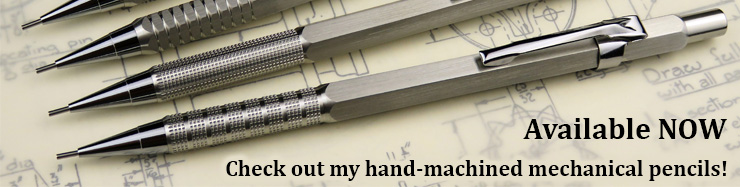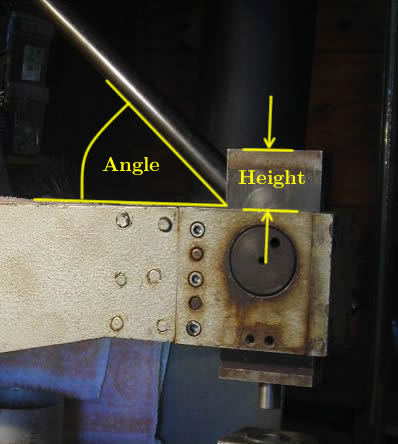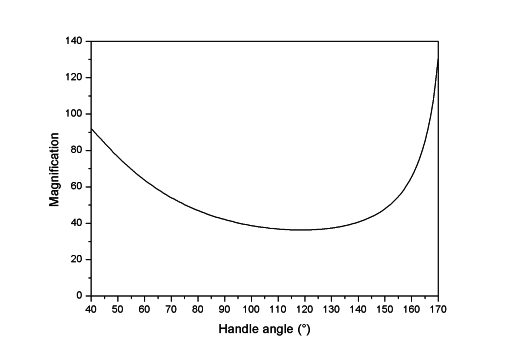﻿ Bench punch leverage measurement# Bench punch leverage measurement (25/07/13)

I've often wondered just what sort of leverage the bench punch has, so decided to do some measurements to find out. The leverage isn't linear, since the punch uses a clever toggle lever arrangement inside the ram.

First, I measured the position of the ram (height above the top of the punch frame) at different handle angles. Here's what I measured:I then fitted a polynomial curve (in Excel) to the data and differentiated it. This gives the rate of change of ram position with handle angle - units of mm per degree. Now, the handle is approximately 55cm long from the pivot to where you'd normally pull. A 55cm radius gives a movement of about 9.6mm at the end per degree of rotation (2*π*550/360). So, if we divide our differentiated curve by 9.6mm, we now have a graph of the rate of change of ram position with handle position, in units of mm of ram travel per mm of handle travel. Finally, to get the "magnification" or leverage, we just take the inverse of this.

Here is an Excel spreadsheet with all of the data, and here is a graph of the magnifcation versus handle angle:You can see how it varies with handle position (because of the toggle lever arrangement inside the ram) but is at least 40x over most of the travel.